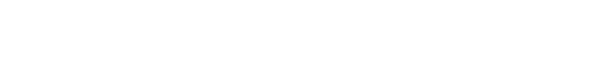# Dart – how to assign int/String values to enum

Dart supports only the simplest case on enum and its index always starts from 0. However, we often want to assign values that might be number or string values. This post shows how to implement it in Dart.

## Basic enum usage

First of all, we need to know how Dart enum works. We can define enum value in this format.

enum MyEnum {
hello,
seven,
}

It is very simple. It doesn’t have any value and we can’t set a value here. When we call enum value it returns the full name of the variable. It looks like a string value but actually, it’s not as you can see in the result below. If we want to compare with string we need to call toString() function.

print(MyEnum.hello);  // MyEnum.hello
print(MyEnum.hello == "MyEnum.hello"); // false
print(MyEnum.hello.toString() == "MyEnum.hello"); // true

Some of you might think enum value returns a number. If we want to use it as a number value we need to use the index getter property. It always starts from 0.

print(MyEnum.hello.index); // 0
print(MyEnum.seven.index); // 1

We often want to assign an arbitrary value to enum. How can we implement enum like other languages? For example, we can define enum like this in TypeScript.

enum MyStringEnum {
hello = "hello";
night = "good night";
}
enum MyNumberEnum {
seven = 7;
nine = 9;
}

How can we implement it in Dart?

## Defining static const values in class

Even if it’s not possible to set the value to enum we can do it in class.

class MyEnum1 {
static String get hello => "Hello";
static int get seven => 7;
}

print(MyEnum1.hello); // Hello
print(MyEnum1.seven); // 7

The data type is obvious and we don’t need additional properties like index or toString() when comparing the value. One point that we should beware of is that we can instantiate the class.

var instance = MyEnum();

It is not so good to be able to instantiate even though it’s useless. Let’s make it abstract. I think it doesn’t necessarily need to be a getter. Let’s change it to normal property.

abstract class MyEnum2 {
static String hello = "Hello";
static int seven = 7;
}

If it is an abstract class Dart compiler shows an error where it is instantiated.

var instance = MyEnum2();
// Abstract classes can't be instantiated.
// Try creating an instance of a concrete
// subtype.dart (instantiate_abstract_class)

## Using map as enum (Not good way)

We shouldn’t use a map as a replacement for enum value because IntelliSense doesn’t work. When we want to change the name we have to replace them carefully because rename function in IDE doesn’t work for the string value.
If we implement it looks like this.

Map<String, dynamic> mapValues = {
"hello": "hello",
"seven": 7,
};
print(mapValues["hello"]);  // hello
print(mapValues["seven"]);  // 7
print(mapValues["undefined"]); // null

Since "undefined" is not defined in the map variable, it returns null.

## Defining extension for enum (Dart >= 2.7)

Another solution is defining an extension. The extension can be used if Dart version is 2.7 or later.

When we want to handle the value as a real enum value this is the only way. If we use static value in class it is no longer enum but string or number. Let’s see an example. We can set our own values.

### Set number to enum

If we want to set a number value the code looks like this.

extension MyEnumExtension on MyEnum {
int get id {
switch (this) {
case MyEnum.hello:
return 1;
case MyEnum.seven:
return 7;
default:
return -1;
}
}
}
print(MyEnum.hello.id); // 1
print(MyEnum.seven.id); // 7

### Set String value to enum

We can set the arbitrary value for each property. What if we want to set string values?

extension MyEnumExtension on MyEnum {
String get value {
switch (this) {
case MyEnum.hello:
return "hello";
case MyEnum.seven:
return "seven";
default:
return "";
}
}
}
print(MyEnum.hello.value); // hello
print(MyEnum.seven.value); // seven

### Value comparison

Do you want to compare values sometimes with the property name, sometimes its value? Yes, we can implement it.

extension MyEnumExtension on MyEnum {
bool isEqual(dynamic value) {
if (value is String) {
return this.toString() == value || this.value == value;
} else if (value is int) {
return this.id == value;
} else {
return false;
}
}
}

I used toString() and value getter because toString() returns full name like "MyEnum.hello" for example. You can of course remove it if it’s not necessary. The result is the following.

print(MyEnum.hello.isEqual("MyEnum.hello")); // true
print(MyEnum.hello.isEqual("hello")); // true
print(MyEnum.hello.isEqual("hello1")); // false
print(MyEnum.hello.isEqual(1)); // true
print(MyEnum.hello.isEqual(2)); // false

We need to write a conditional clause to get the target value. I used switch-case and wrote the default keyword but it seems to have no case to reach there. I tried the following code

print(("hello" as MyEnum).id);

but an unhandled exception occurred at runtime.

Unhandled exception:
type 'String' is not a subtype of type 'MyEnum' in type cast
#0      EnumTest.execute (...blogpost-dart/src/one-file/EnumTest.dart:26:20)
#1      main (...blogpost-dart/src/main.dart:10:12)
#2      _delayEntrypointInvocation.<anonymous closure> (dart:isolate-patch/isolate_patch.dart:283:19)
#3      _RawReceivePortImpl._handleMessage (dart:isolate-patch/isolate_patch.dart:184:12)

## Overview

Basic Enum support only the simplest case. If we need to assign value we need other ways. The possible solutions are following.

• Defining static value in class
• Defining extension

A class can handle arbitrary variables but they are normal values rather than enum. If we really want to use enum value, defining extension is the only way to go. In this way, we can set whatever we want to the property and handle them in the same way as other languages. It depends on your case which solution to choose.
Choose extension if you need to compare values. Otherwise, static value is enough.

Articles that you may want to read.